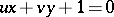# Tangential coordinates

(diff) ← Older revision | Latest revision (diff) | Newer revision → (diff)
A term for the coefficients in the equation of a straight line, regarded as coordinates. In the equation of a straight line, the coefficientsandare called non-homogeneous tangential coordinates. In the homogeneous equation of the straight line,, the coefficients are called homogeneous tangential coordinates. The equation linking the tangential coordinates of the tangent to the curve is called the tangential equation of this curve. The tangential equation of an algebraic curve is algebraic. The tangential equation of a curve is dual to the equation in point coordinates. The degree of the tangential equation is called the class of the curve.